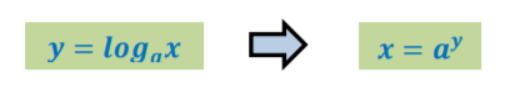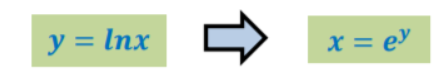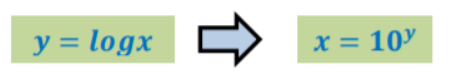# Logarithmic Functions

 Would You Rather Listen to the Lesson?In precalculus, students learn about functions, including logarithmic functions. They learn what logarithmic functions represent and acquire the necessary skills to evaluate such functions and find their domain and range.

It’s no secret that logarithms can be challenging to master. Luckily, there are tons of tips that math teachers can use to help out students who are struggling with logarithms. Apply the tips from this article in your classroom and never worry about teaching logarithmic functions again!

## Tips on Teaching Logarithmic Functions

### Exponent Vs. Logarithm

A good way to start your lesson on logarithmic functions is by reviewing exponents. Students already know that exponents are small numbers, like superscripts, written at the upper right of a number or a variable (a letter). They also know how to take exponents.

For instance, ask students what does it mean if we say 2 to the fourth power, or 24? They should be able to quickly tell you that 24 is the same as 2 × 2 × 2 × 2, as we know that exponents represent repeated multiplication. That is, 24 = 16 and 2 × 2 × 2 × 2 = 16.

Now ask students to think of things in a different way. Point out that we know that we get to 16 when we raise 2 to some power, but let’s say we don’t know what that power is. For example, you can write it in this way on the whiteboard, by marking this unknown power with x:

2x = 16

In this case, we know that the unknown power or x would have to be 4. Highlight that this is what logarithms are basically about, figuring out what power we have to raise two so that you get another number.

Add that we can also write the previous example of 2x = 16 as log216 = x (“log base 2 of 16”), as these are equivalent statements. The first one says: “if I take 2 and raise it to x power I get 16”, and the second one asks: “what power do I need to raise 2 to, to get 16 and this will equal x?”### What Is a Logarithm?

Now you can proceed with defining logarithms. You can simply say that a logarithm is an exponent i.e. logax (“log base a of x”) is the exponent to which a must be raised to get x. You can present this in the following manner:Where a > 0, a ≠ 1 and x > 0.

In simple terms, logarithms are the opposite of exponentials, as they basically “undo” exponentials. You can also use this video by Khan Academy in your class, which contains a simple introduction on logarithms, including examples.

#### Types of Logarithms

Explain to students that there are several types of logarithms, according to the different values of the base of the given logarithm. However, certain bases are used more frequently. This includes the natural logarithms and the common logarithms.

• Natural logarithm

A natural logarithm () is a special logarithmic function with base = 2.7183. Point out that the regular notation is logex, but we also use a special notation for natural logarithms. You can present it like this:• Common logarithm

A common logarithm () is a special logarithmic function with base = 10. Point out that the regular notation is log10x, but we also use a special notation for common logarithms. You can present it in the following manner:### Evaluating Logarithmic Functions

Explain to students that evaluating logarithmic functions simply means finding the exponent to which a must be raised to get x in logax. Provide an example and show students how we can find this exponent. For instance, let’s say we have the following logarithm:

Log381 = x

What is the value of this logarithm? Explain to students that by applying what we have learned thus far, this is equal to the power we have to raise 3 to so as to get to 81. So we can restate this equation in the following way:

3x = 81

To find x, add that we can experiment a bit. We know that 3 to the first power is 3, 3 to the second power is 9, 3 to the third power is 27, and 3 to the fourth power is 27 multiplied by 3, which is equal to 81. So x is equal to 4. We can write it like this:

Log381 = 4

Use this video in your class to introduce evaluating logarithms. The video starts with simple examples of how we evaluate logs, such as log28 = x. Then, proceed with this video to introduce more advanced examples of evaluating logarithms, such as log82 = x.

### Domain and Range

Once you’ve explained what logarithms are and how we can evaluate them, explain to students how to find the domain and range of logarithmic functions. Students know that logarithms are the inverse of exponentials; thus, logarithmic functions are the inverse of exponential functions.

Point out that the log of zero or a negative number is always undefined, so the domain of f(x) = logax is (0, +∞) and the range is (−∞, +∞). So the domain of a logarithmic function comprises real numbers bigger than 0 and the range comprises real numbers.

Play this video to provide an example. The video contains simple instructions on how to find the domain and range of a given logarithmic function, such as f(x) = log2(x + 4) + 3.

### Asymptote and Intercept

Explain that the asymptote of the logarithmic function is a vertical line that touches the logarithmic function. Intercept is the point where the graph of the logarithmic function meets the x-axis.

Use this video to provide an example of finding the vertical asymptote and x-intercepts of a given logarithmic function, such as y = – log3x + 2.

## Activities to Practice Logarithmic Functions### Log to Exponent Game

This game will help students improve their skills for recognizing that a logarithm is simply an exponent, and their ability to quickly transform a logarithm into its equivalent exponential statement.

To implement this activity in your classroom, you will need red and green construction paper, scissors, and a marker. Create task cards on the construction paper, by writing one logarithm on each card and a matching exponential equation on the red card.

Divide students into small groups of 3, 4 and hand out the cards. The cards remain face down until you give students the signal to start. Explain to students that they need to match the logarithmic statement on a green card with the equivalent exponential statement on a red card.

For example, if there is a green card with the following logarithmic statement: log381 = 4, students must search for a red card with the equivalent exponential statement to match them. That is, they need to search for the red card with 34 = 81 written on it.

Provide a few minutes for students to complete the matching. You can also use a timer for this. Once the time is up, check the matched cards in each group (or designate a ‘checker’ in each group). The group with the greatest number of correctly matched cards wins the game.

### Evaluating Exponents Game

This game is a fun way to help students reinforce their skills to quickly evaluate exponents. To implement the game in your classroom, you’ll need construction paper, scissors, a marker, and several timers.

Draw several task cards on the paper and cut them out. You can also decide to laminate them if you wish to re-use them next year. Write one log question on each card, such as log381 = x, where students need to find the x. Write the answer on the card as well, that is, 4 in this case.

Create several sets of such cards, with each set containing around 20 cards. Pair students up and hand out a set of cards to each pair, as well as a timer. Explain the rules of the game. The set remains face down throughout the game.

Player 1 withdraws one card and reads out the log to player 2, asking them to evaluate it. Player 2 has a few minutes to perform this, while player 1 sets the timer (ex: 2 min or 5 min depending on the difficulty of the game). Then, player 1 draws a card and the process is repeated.

For each correct answer, students earn 1 point. If they answer incorrectly, they lose 2 points. Students keep track of the points they earned throughout the game. In the end, they compare their scores. The player that ends up with the highest score wins the game.

### Group Work

This is a simple activity that will help students apply what they have learned on logarithmic functions and reinforce their knowledge. To implement this activity in your classroom, print out this Assignment Worksheet (Members Only).

Divide students into small groups and hand out the worksheets (one copy per student). Explain to students that the worksheet contains several log-related exercises where they will have to evaluate logs, or determine the domain, range, intercept, and asymptote of logarithmic functions.

Students work together with the members of their group to solve the exercises. Provide some 15 minutes for this. In the end, go through the exercises together and open space for discussion and reflection within the class.

## Before You Leave…

If you enjoyed these tips and activities for teaching logarithmic functions, you may want to check out our lesson that’s dedicated to this topic. So if you need guidance to structure your class and teach pre-calculus, make sure to sign up for more free resources here!

You can also sign up for our membership MathTeacherCoach or head over to our blog – you’ll find plenty of awesome resources for kids of all ages!